# DAV Class 5 Maths Chapter 7 Worksheet 7 Solutions

The DAV Maths Book Class 5 Solutions and DAV Class 5 Maths Chapter 7 Worksheet 7 Solutions of Multiplication and Division of Decimal Numbers offer comprehensive answers to textbook questions.

## DAV Class 5 Maths Ch 7 Worksheet 7 Solutions

Question 1.
Divide the following:
(a) 0.5 ÷ 2
Solution:
0.5 ÷ 22
Dividend = 0.5
Divisor = 2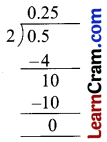Q = 0.25
R = 0

(b) 3.4 ÷ 4
Solution:
3.4 ÷ 4
Dividend = 3.4
Divisor = 4Q = 0.85
R = 0(c) 12.6 ÷ 5
Solution:
12.6 ÷ 5
Dividend = 12.6
Divisor = 5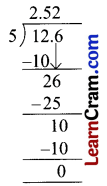Q = 2.52
R = 0

(d) 6.05 ÷ 25
Solution:
6.05 ÷ 25
Dividend = 6.05
Divisor = 25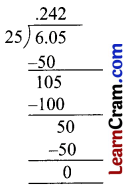Q = 0.242
R = 0

(e) 11.7 ÷ 6
Solution:
11.7 ÷ 6
Dividend = 11.7
Divisor = 6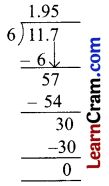Q = 1.95
R = 0(f) 12.06 ÷ 12
Solution:
12.06 ÷ 12
Dividend = 12.06
Divisor = 12Q = 1.005
R = 0

(g) 9.2 ÷ 16
Solution:
9.2 ÷ 16
Dividend = 9.2
Divisor = 16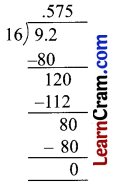Q = 0.525
R = 0

(h) 3.75 ÷ 6
Solution:
3.75 ÷ 6
Dividend = 3.75
Divisor = 6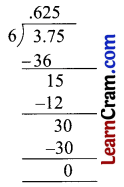Q = 0.625
R = 0(i) 8.5 ÷ 17
Solution:
8.5 ÷ 17
Dividend = 8.5
Divisor = 17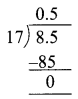Q = 0.5
R = 0

(j) 14.4 ÷ 12
Solution:
14.4 ÷ 12
Dividend = 14.4
Divisor = 12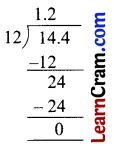Q = 0.9
R = 0